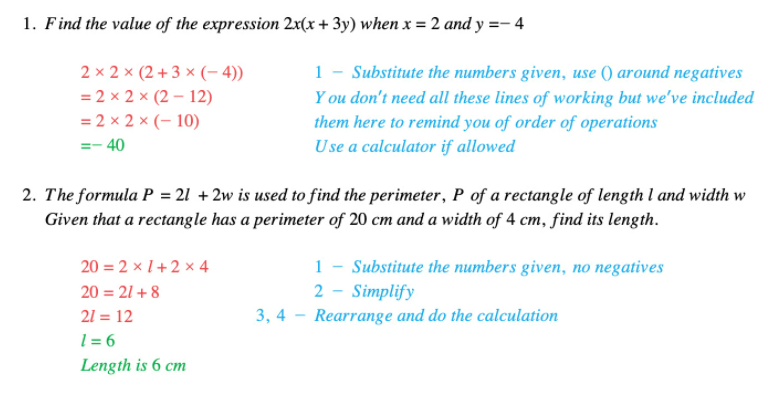# Edexcel IGCSE Maths 复习笔记 2.9.1 Substitution

Edexcel IGCSE Maths 复习笔记 2.9.1 Substitution

#### What is substitution?

• Substitution is where we replace letters in a formula with their values
• This allows you to find one other value that is in the formula

#### How do we substitute?

• Write down the FORMULA if not clearly stated in question
• SUBSTITUTE the numbers given – use ( ) around negative numbers
• SIMPLIFY if you can
• REARRANGE if necessary – it is usually easier to substitute first
• Do the CALCULATION – use a calculator if allowed

#### Worked Example